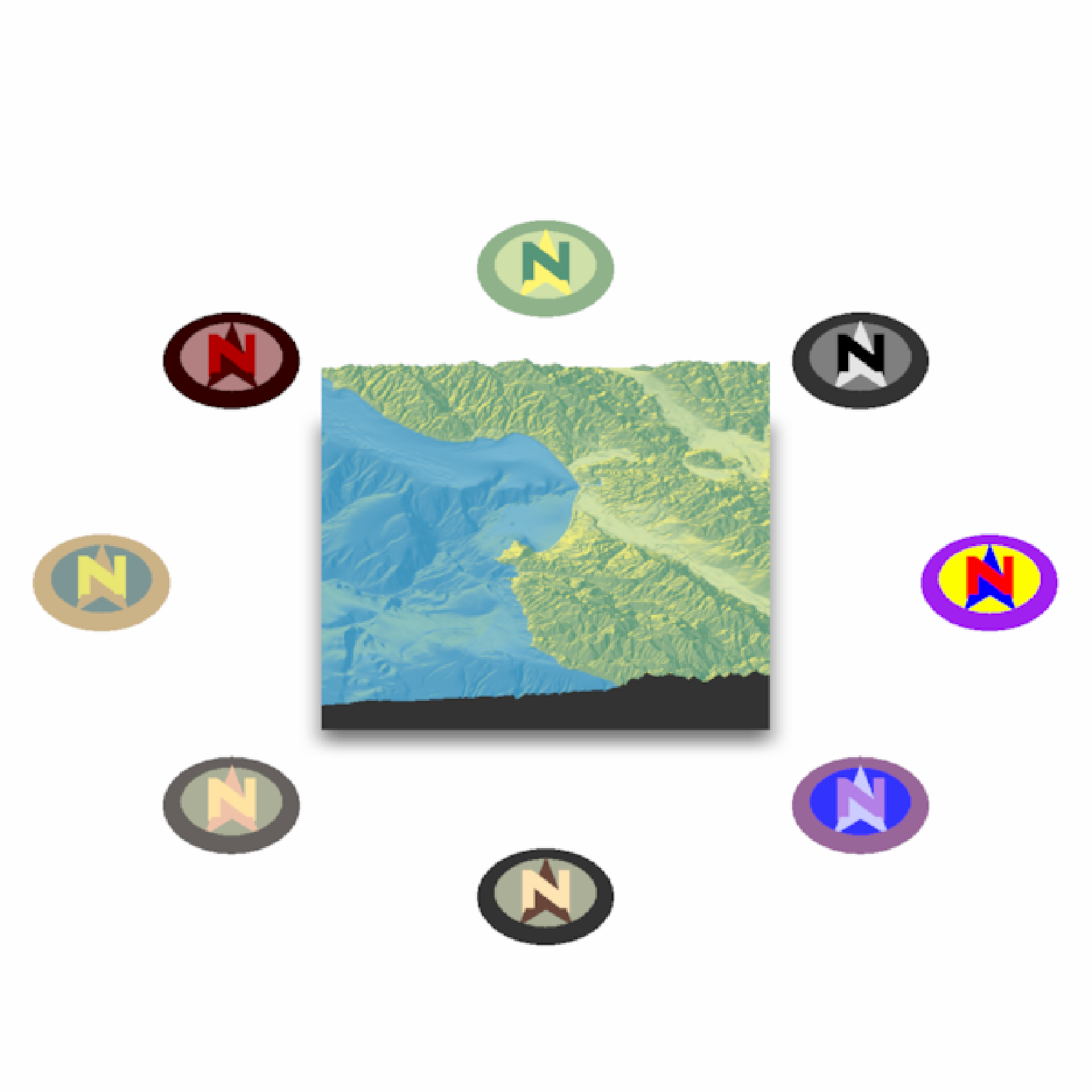Places a compass on the map to specify the North direction.

render_compass(
angle = 0,
position = "SE",
altitude = NULL,
zscale = 1,
x = NULL,
y = NULL,
z = NULL,
scale_distance = 1,
color_n = "darkred",
color_arrow = "grey90",
color_background = "grey60",
color_bevel = "grey20",
position_circular = FALSE,
clear_compass = FALSE
)

## Arguments

angle

Default 0. The direction the arrow should be facing.

position

Default SE. A string representing a cardinal direction. Ignored if x, y, and z are manually specified.

altitude

Default NULL. Altitude of the compass, defaults to maximum height in the map.

zscale

Default 1. The ratio between the x and y spacing (which are assumed to be equal) and the z axis. Only used in combination with altitude.

x

Default NULL. X position. If not entered, automatically calculated using position argument.

y

Default NULL. Y position. If not entered, automatically calculated using position argument.

z

Default NULL. Z position. If not entered, automatically calculated using position argument.

Default NULL. The radius of the compass. If not entered, automatically calculated. Increase or decrease the size of the compass.

scale_distance

Default 1. Multiplier that moves the compass away from the center of the map.

color_n

Default darkred. Color of the letter N.

color_arrow

Default grey90. Color of the arrow.

color_background

Default grey20. Color of the area right under the arrow.

color_bevel

Default grey20. Color of the bevel.

position_circular

Default FALSE. If TRUE, will place compass at a constant radius away from the map, as opposed to directly next to it. Overridden if user manually specifies position.

clear_compass

Default FALSE. Clears the compass symbol(s) on the map.

## Value

Adds compass to map. No return value.

## Examples

#Add a North arrow to the map, by default in the bottom right (SE)
if(run_documentation()) {
montereybay %>%
plot_3d(montereybay,theta=-45, water=TRUE)
render_compass()
render_snapshot()
}
#> montereybay dataset used with no zscale--setting zscale=50.  For a realistic depiction, raise zscale to 200.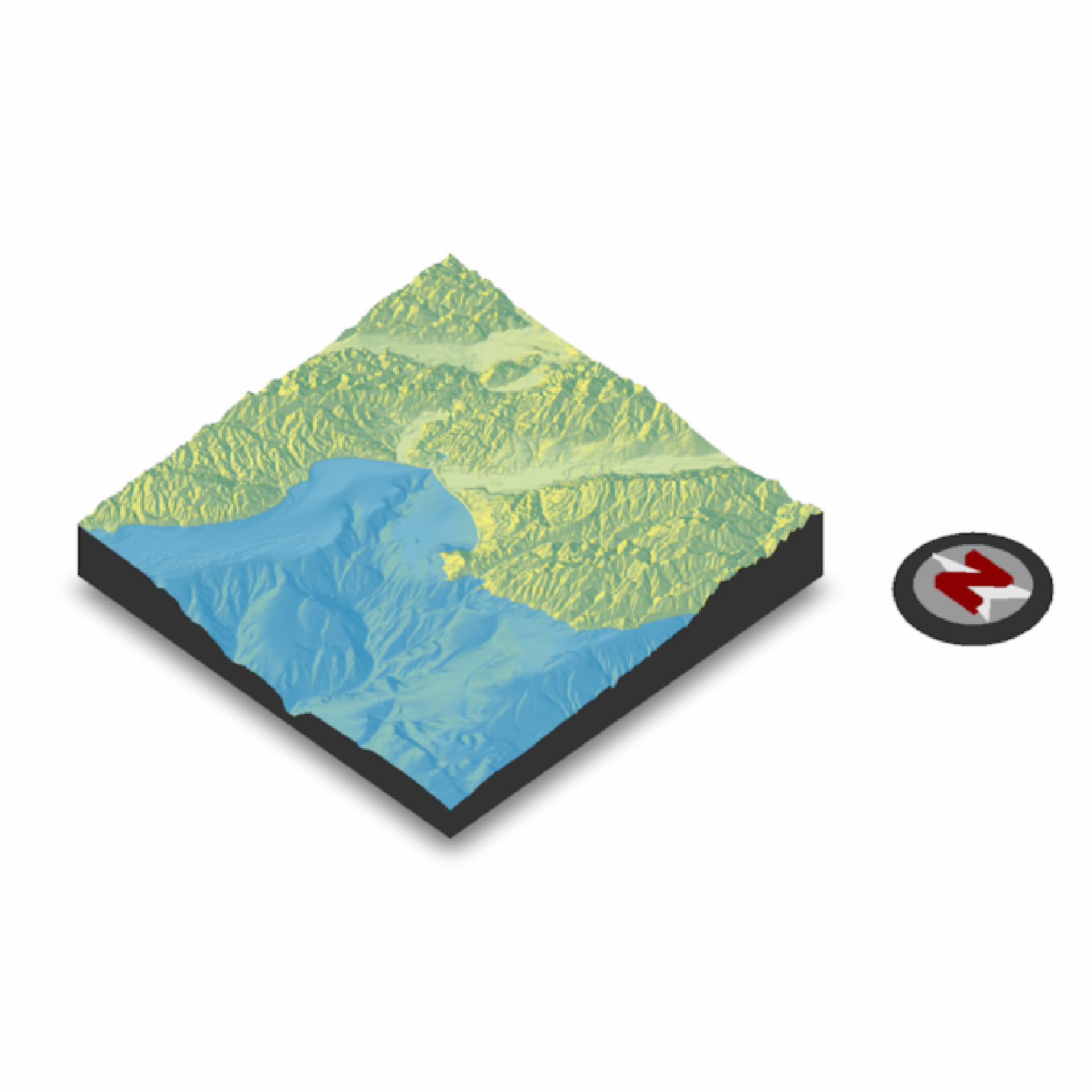if(run_documentation()) {
#Remove the existing symbol with clear_compass = TRUE
render_compass(clear_compass = TRUE)

#Point the N towards the light, at 315 degrees:
render_compass(angle = 315)
render_snapshot()
}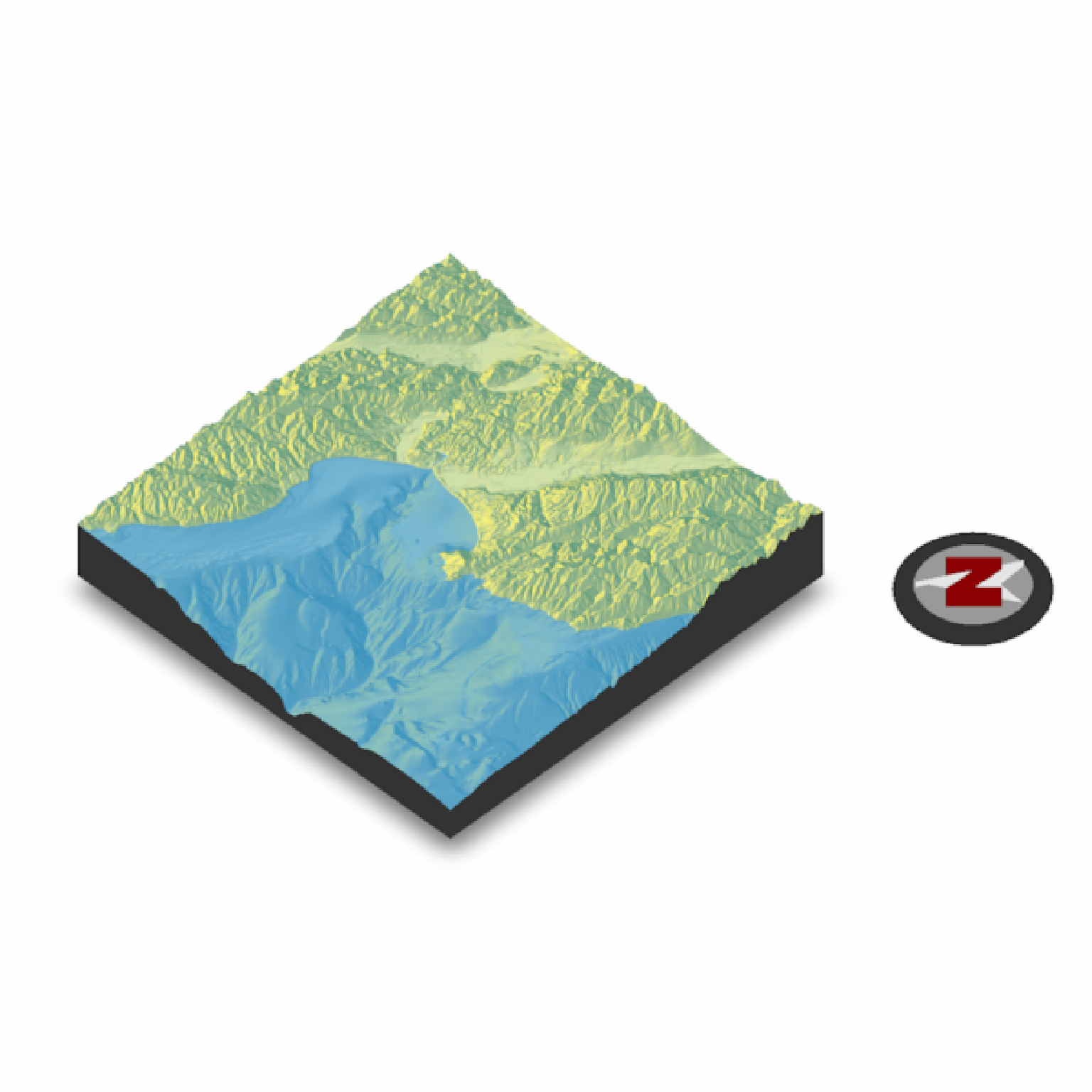if(run_documentation()) {
render_compass(clear_compass = TRUE)

#We can change the position by specifying a direction (here are three):
render_camera(theta=45,phi=45)
render_compass(position = "NW")
render_compass(position = "E")
render_compass(position = "S")
render_snapshot()
}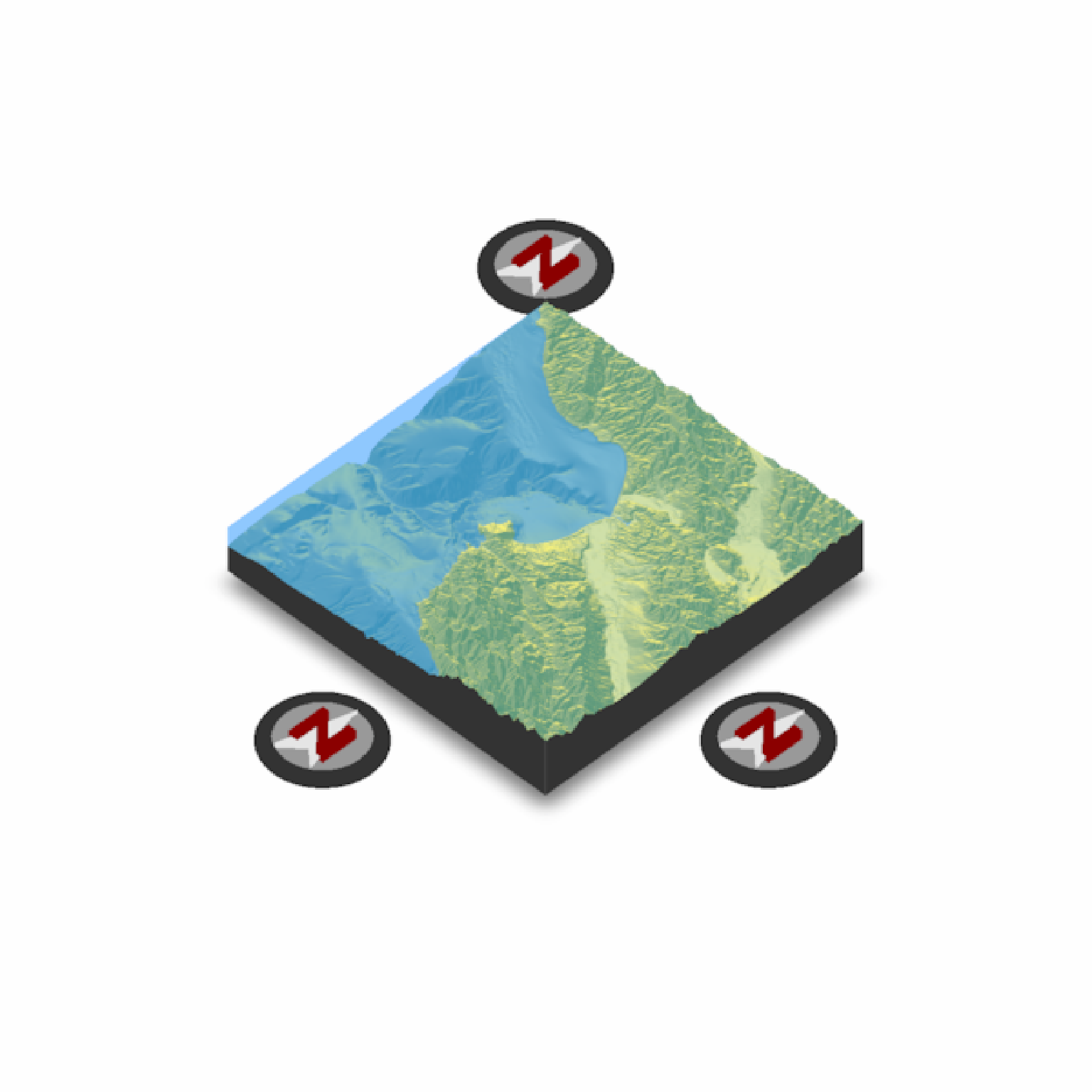if(run_documentation()) {
render_compass(clear_compass = TRUE)

#We can also change the distance away from the edge by setting the scale_distance argument.
render_compass(position = "NW", scale_distance = 1.4)
render_compass(position = "E", scale_distance = 1.4)
render_compass(position = "S", scale_distance = 1.4)

#Zoom in slightly:
render_camera(theta=45,phi=45,zoom=0.7)
render_snapshot()
}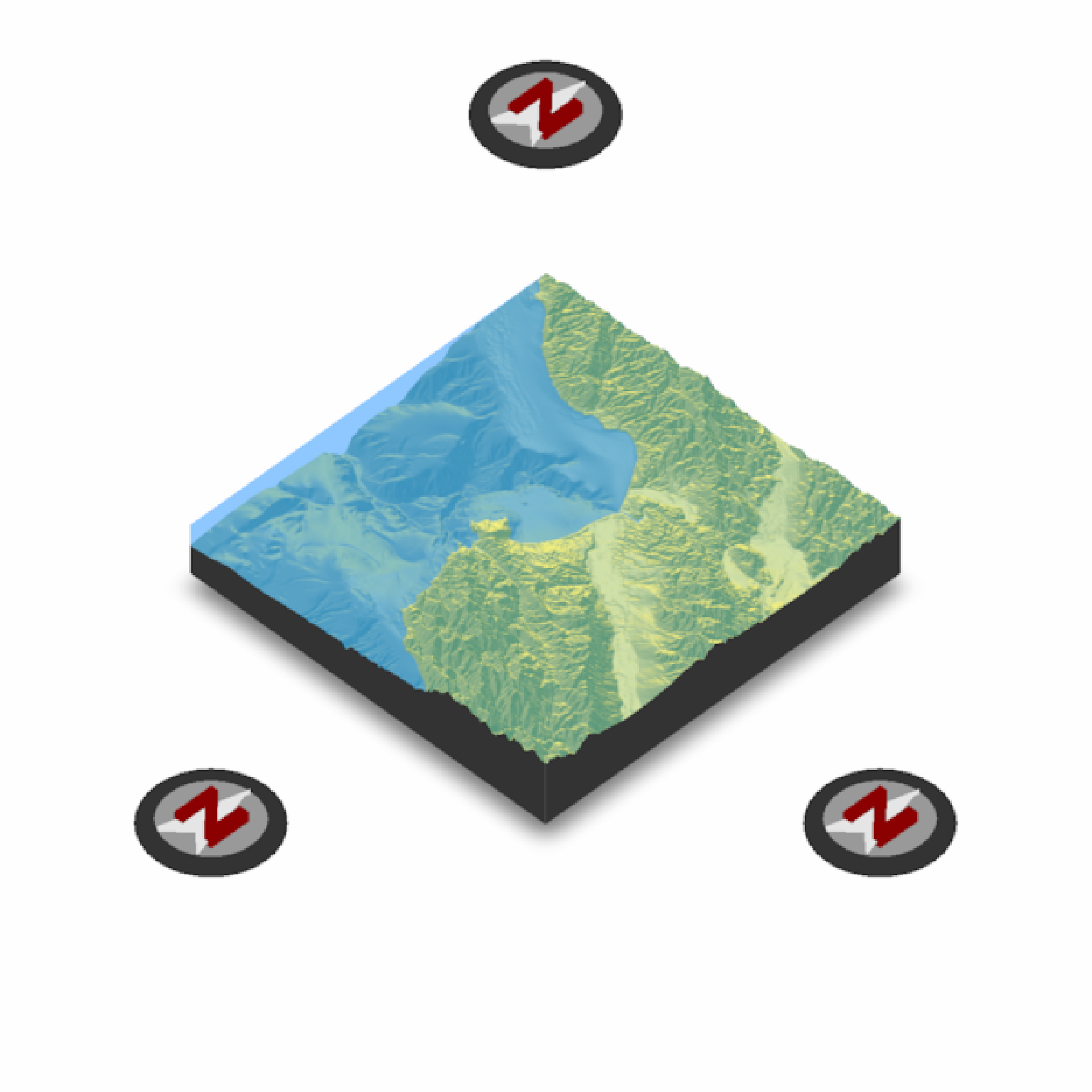if(run_documentation()) {
render_compass(clear_compass = TRUE)

#We can also specify the radius directly with compass_radius:
render_camera(theta=0,phi=45,zoom=1)
render_compass(position = "N", scale_distance = 1.5, compass_radius=200)
render_compass(position = "E", scale_distance = 1.4, compass_radius=50)
render_compass(position = "S", scale_distance = 1.3, compass_radius=25)
render_compass(position = "W", scale_distance = 1.2, compass_radius=10)
render_snapshot()

render_compass(clear_compass = TRUE)
}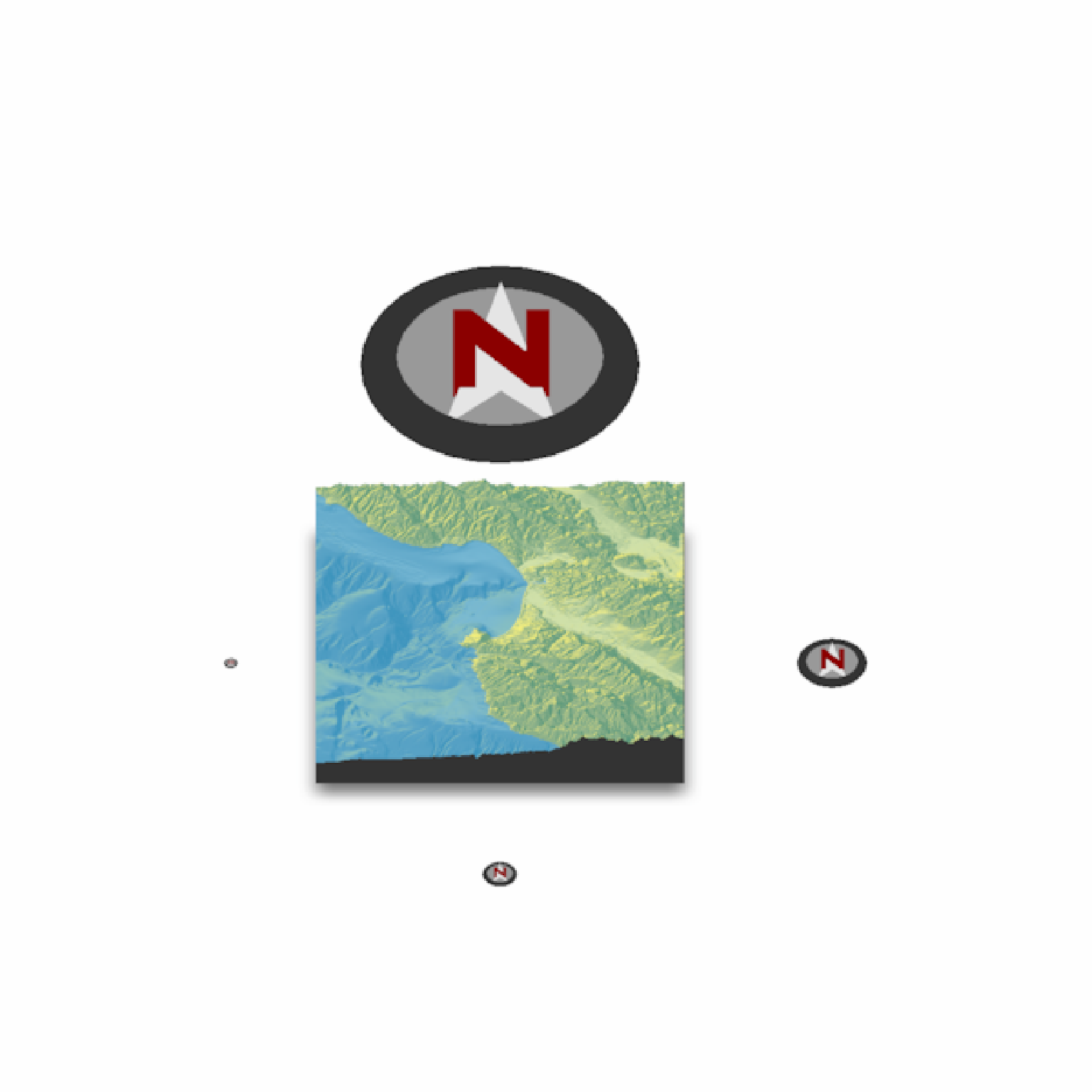if(run_documentation()) {
#We can also adjust the position manually, be specifying all x, y and z arguments.
render_camera(theta=-45,phi=45,zoom=0.9)
render_compass(x = 150, y = 50, z = 150)
render_snapshot()
}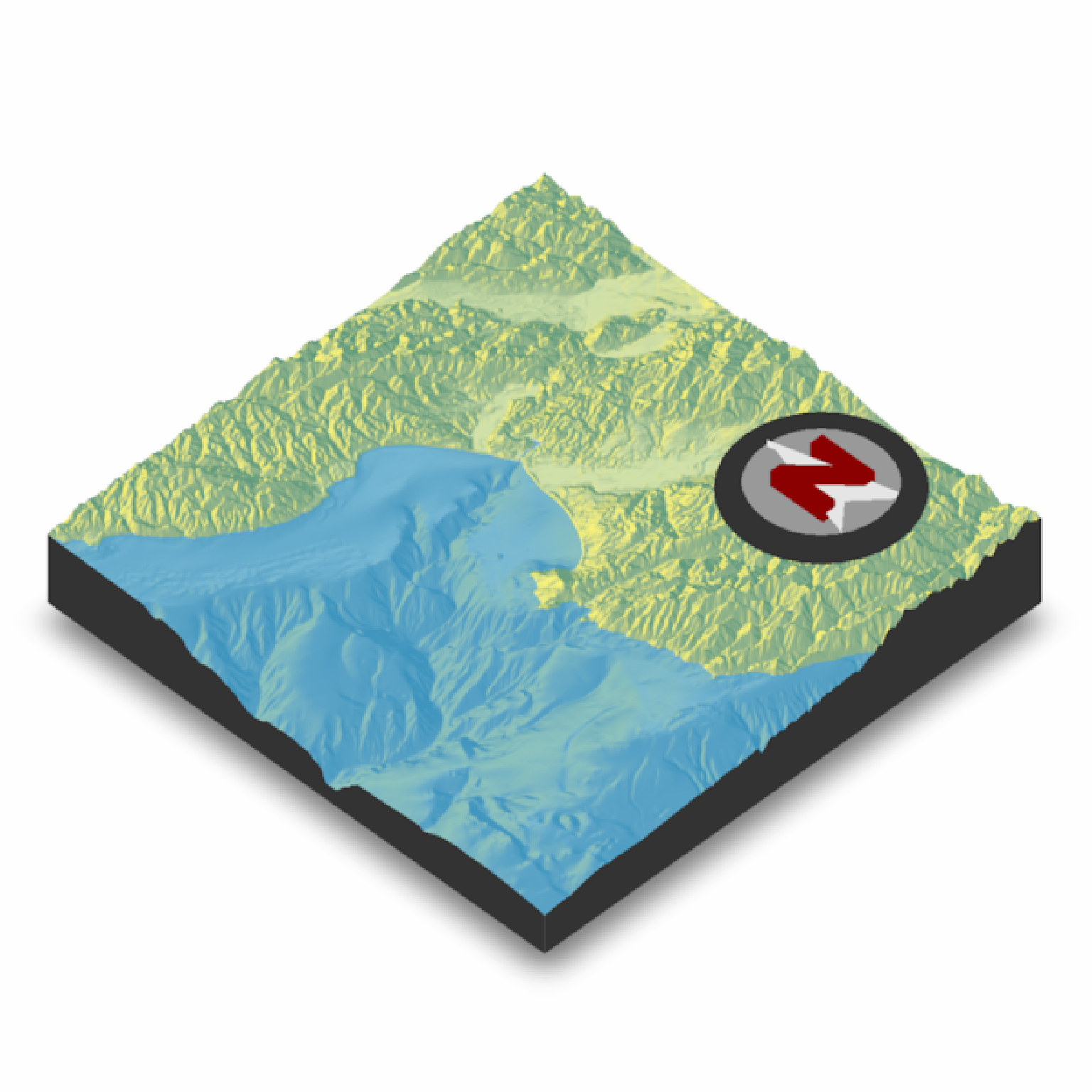if(run_documentation()) {
# Compass support is also included in render_highquality()
render_highquality(clamp_value=10, min_variance = 0, sample_method = "sobol_blue")
}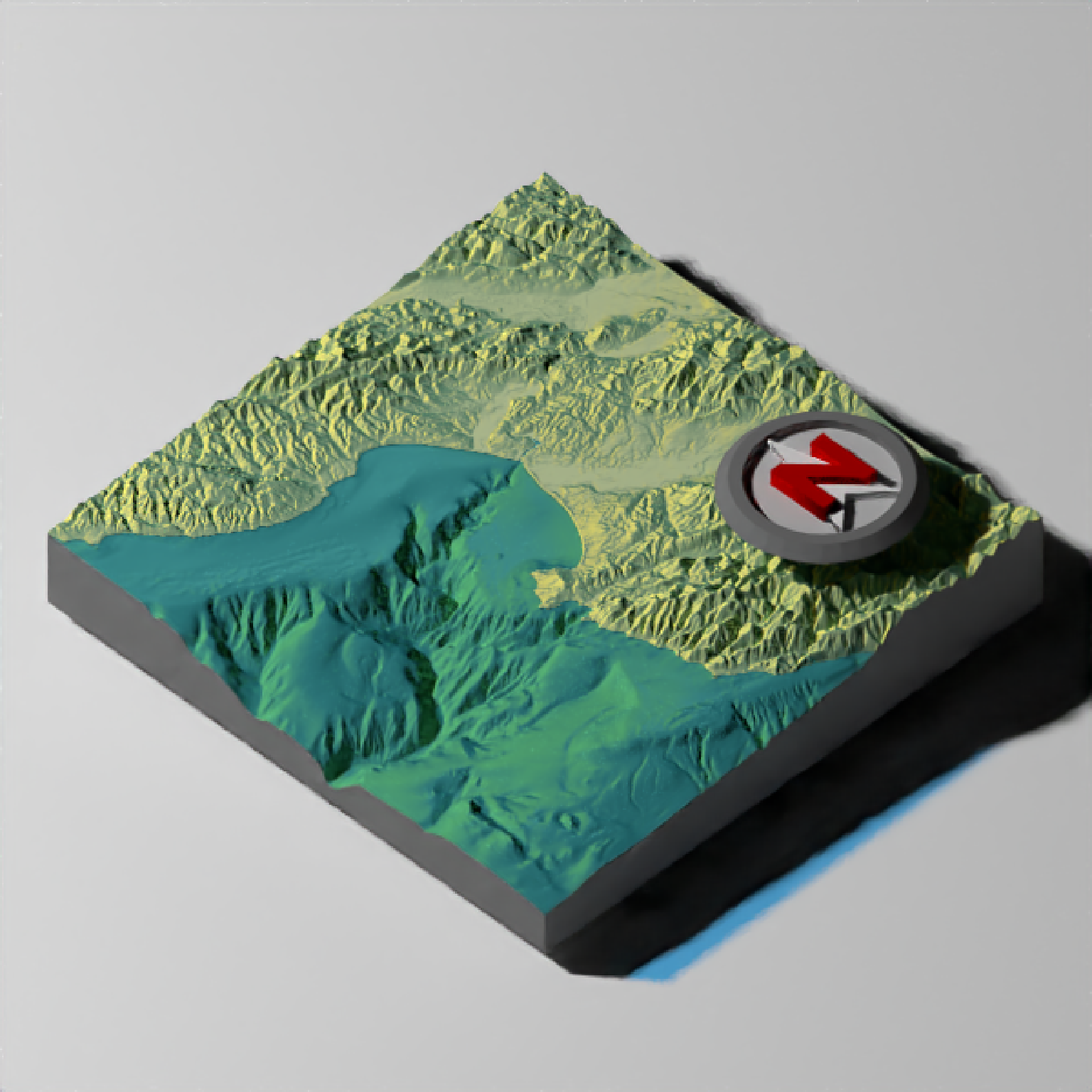if(run_documentation()) {
render_compass(clear_compass = TRUE)

#We can change the colors in the compass, and also set it a constant distance away with
#position_circular = TRUE:

render_camera(theta=0,phi=45,zoom=0.75)
render_compass(position = "N", color_n = "#55967a", color_arrow = "#fff673",
color_background = "#cfe0a9", color_bevel = "#8fb28a", position_circular = TRUE)
render_compass(position = "NE", color_n = "black", color_arrow = "grey90",
color_background = "grey50", color_bevel = "grey20", position_circular = TRUE)
render_compass(position = "E", color_n = "red", color_arrow = "blue",
color_background = "yellow", color_bevel = "purple", position_circular = TRUE)
render_compass(position = "SE", color_n = c(0.7,0.5,0.9), color_arrow = c(0.8,0.8,1),
color_background = c(0.2,0.2,1), color_bevel = c(0.6,0.4,0.6),
position_circular = TRUE)
render_compass(position = "S", color_n = "#ffe3b3", color_arrow = "#6a463a",
color_background = "#abaf98", color_bevel = "grey20", position_circular = TRUE)
render_compass(position = "SW", color_n = "#ffe3a3", color_arrow = "#f1c3a9",
color_background = "#abaf98", color_bevel = "#66615e", position_circular = TRUE)
render_compass(position = "W", color_n = "#e9e671", color_arrow = "#cbb387",
color_background = "#7c9695", color_bevel = "#cbb387", position_circular = TRUE)
render_compass(position = "NW", color_n = c(0.7,0,0), color_arrow = c(0.3,0,0),
color_background = c(0.7,0.5,0.5), color_bevel = c(0.2,0,0), position_circular = TRUE)
render_snapshot()
}